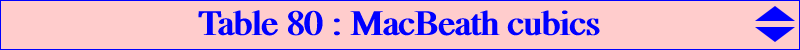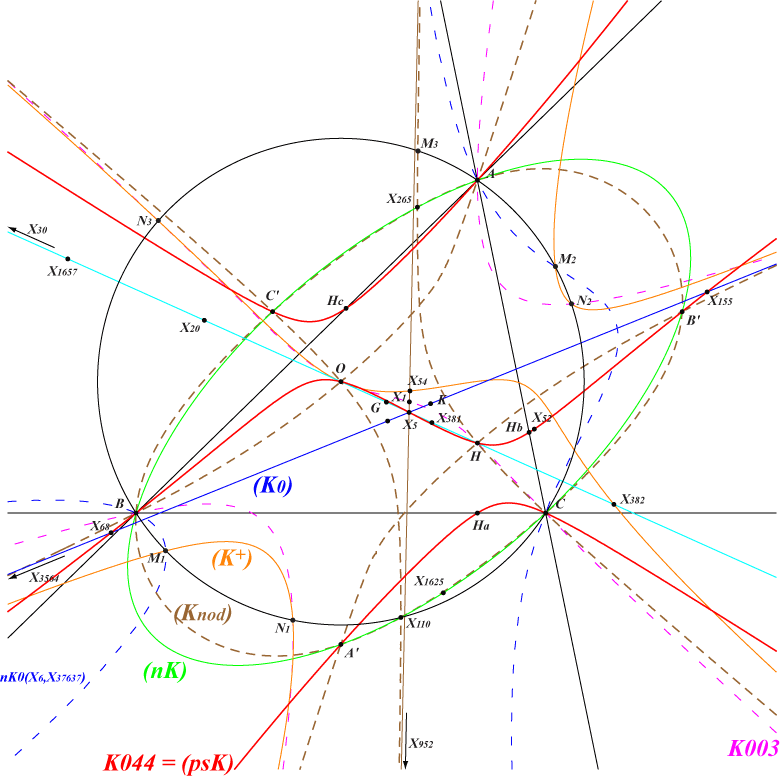A MacBeath cubic is a circum-cubic which passes through the four foci of the MacBeath inconic, namely O, H and two imaginary isogonal points on the perpendicular bisector of OH and on the circum-conic passing through X(54), X(110) which is its isogonal transform. Every MacBeath cubic MB(P) is a spK(P, X5) for some point P. See CL055 for spK cubics. All these cubics are in a same net which contains lots of remarkable cubics. Some of these cubics are already mentioned in Table 54, see line Q = X(5) in the table and note 3. These are the cubics obtained when P lies on the Euler line. This page presents a more in-depth study. Recall that MB(P) passes through the isogonal conjugate P* of P and the reflection P' of P in X(5). MB(P), pK(X6, P) share the same points at infinity and MB(P), pK(X6, P') share the same points on (O). MB(P) and MB(P') are isogonal transforms of one and another. They coincide if and only if P = X(5) giving the Napoleon cubic K005, or P lies on the line at infinity, giving the focal isogonal nKs mentioned below. More generally, for every P on K005, MB(P) passes through P and obviously also through P*, also on K005, and P'.MB(P) is an equilateral cubic if and only if P = O, giving the McCay stelloid K028 = psK(X4, X264, X3).   MB(P) is a circular cubic if and only if P lies on the line at infinity. In this case, it is an isogonal focal nK with singular focus on the circumcircle. Its root lies on the trilinear polar of X(264), passing through X(297), X(525), X(850), X(2501), X(2592), X(2593), etc. Every cubic is the locus of foci of inscribed conics centered on a line passing through X(5). K164 is the only nK0 of this type.   MB(P) is a K0 (no term in xyz) if and only if P lies on the (blue) line passing through X(5), X(6) and many other centers.   MB(P) is a psK if and only if P lies on K044 = pK(X216, X4), the Darboux cubic of the orthic triangle. In this case, the pseudo-pole Ω lies on K176 = pK(X32, X4) and the pseudo-pivot Q lies on K674 = pK(X324, X264). It follows that MB(P) is a pK if and only if P is X(5), X(68), X(155) corresponding to the Napoleon cubic K005, K1318 = pK(X571, X4), K1337 = pK(X2165, X847) respectively (see yellow cells in the table below).P X(3) X(4) X(5) X(52) X(68) X(155) X(5562) X(8800) X(8905) X(8906) X(34428) X(58700) X(58701) Ω X(4) X(184) X(6) X(3) X(571) X(2165) X(25) X(155) X(39109) X(39110) X(39111) X(39112) X(58702) Q X(264) X(2) X(5) X(311) X(4) X(847) X(324) X(39113) X(39114) X(39115) X(39116) X(58703) X(39117) psK K028 K009 K005 K1338 K1318 K1337 K1339MB(P) is a nK if and only if P lies on the line at infinity as above, or on the (green) circum-conic with center X(5), perspector X(216), called the Johnson circum-conic. In this case, its pole Ω lies on the nodal cubic cK(#X6, X4) and its root lies on the circum-conic with perspector X(5). When P is A, B, C, X(110), X(265), the nK decomposes into a line and a conic. When P is a reflection A', B', C' of A, B, C in X(5), the nK is a nodal cubic with node A, B, C respectively. The isogonal transform of spK(A', X5) is a rectangular hyperbola with center X(5), passing through A, A', the four foci of the MacBeath inconic, the infinite points of the A-bisectors.   MB(P) is a K+ if and only if P lies on a (orange) cubic passing through X(3), X(54), X(382), X(15801), the infinite points of K005, the points on (O) of K003 (vertices N1, N2, N3 of the CircumNormal triangle) and on nK0(X6, X37367). These latter points are the antipodes M1, M2, M3 of the points of pK(X6, X1657) on (O), where X(1657) is the reflection of X(3) in X(20). This cubic meets K005 again at six finite points on the rectangular hyperbola passing through X(3), X(20), X(54), X(155), X(2574), X(2575).   MB(P) is a nodal cubic if and only if P lies on a (dashed brown) very complicated bicircular curve of degree 12, passing through X(3), X(4), X(110), X(265), X(952), the infinite points of the lines passing through X(5) and the excenters, the infinite points of the circum-conic with perspector X(577). This curve is symmetric about X(5).   The table below shows all the listed cubics and several other remarkable examples. F is the singular focus of a focal cubic. ∞Knnn are the infinite points of Knnn.P cubic type X(i) on the curve for i = remarks X(5) K005 pK see the page ∞K005 X(4) K009 nodal psK vertices of the CircumNormal triangle, see the page ∞K006 X(3) K028 nodal psK+ see the page stelloid, ∞K003 X(542) K072 focal nK 2, 3, 4, 6, 542, 842, 6328, 14246, 14355, 14356, 14357, 14366, 38940 F = X(842) X(3564) K164 focal nK0 3, 4, 468, 895, 3563, 3564, 6337, 14248, 51847 F = X(3563) X(952) K165 focal nK 1, 3, 4, 952, 953, 3109, 6790, 14260, 14887, 36944, 38941, 43692, 52200 strophoid, F = X(953) X(2782) K166 focal nK 3, 4, 1316, 2698, 2782, 9513, 14251, 14382, 39641, 39642, foci of Brocard inellipse F = X(2698) X(30) K187 focal nK 3, 4, 30, 74, 34209, 34210, 39162, 39163, 39164, 39165, 42411, 42412, 46357, 46358, 51898, 51899, foci of Steiner inellipse F = X(74), ∞K001 X(511) K433 focal nK 3, 4, 23, 32, 67, 76, 98, 511, 43087, 52179 F = X(98) X(54) K526 central 3, 4, 5, 54, 6288, 25043 center = X(5) X(6) K527 2, 3, 4, 76, 1352, 1689, 1690, 5486, 14355, 14376, 34511, 36823, 43084, 51259, 51260 ∞K102 X(381) K759 2, 3, 4, 3431, 7607, 9716, 9717, 14355, vertices of the Thomson triangle X(2) K762 3, 4, 6, 381, 576, 9214, 14356, 15274, 39239 ∞K002 X(1352) K835 3, 4, 6, 32, 1995, 3425, 8743, 14356, vertices of the Grebe triangle X(20) K846 3, 4, 64, 382, 3357, 51342, 52130 ∞K004 X(382) K850 central 3, 4, 20, 11270, 38942, antipodes of A, B, C on (O) center = X(3) X(523) K932 focal nK 3, 4, 110, 523, 7471, 14264, 15453, 15454 F = X(110) X(1154) K1154 focal nK 3, 4, 1141, 1154, 2070, 25043, 25044, 33565, 38896, 38897 F = X(1141) X(32423) K1180 focal nK 3, 4, 5, 54, 14979, 32423, 38539, 38542 F = X(14979) X(512) K1282 focal nK 3, 4, 99, 512, 7468, 14265, 34157, 51478, 51479, 51480 F = X(99) X(68) K1318 pK 3, 4, 24, 155, 1147, 2904, 15317, 34756 X(1625) K1319 nK 2, 3, 4, 879, 14355, 14592, 15412, 43665 X(155) K1337 pK 3, 4, 68, 254, 847 / isogonal transform of K1318 X(52) K1338 psK 3, 4, 76, 96, 1147, 2888 / isogonal transform of K1339 X(5562) K1339 psK 3, 4, 32, 52, 847 / isogonal transform of K1338 X(355) K1340 1, 3, 4, 56, 3417, 10571, 11101, 15446, 17104 / isogonal transform of K1345 X(2888) K1341 3, 4, 195, 3432, 3459, 10274, 13621, 25044, 38539, 42059 / isogonal transform of K1342 X(195) K1342 3, 4, 195, 2888, 3459, 13418, 25043, 38542 / isogonal transform of K1341 X(58922) K1343 3, 4, 5, 49, 54, 1147, 3432, 10274, 58923, 58924, 58925 X(18436) K1344 3, 4, 24, 847, 5889, 16000, 25044, 59274, 59275, 59276, 59277, 59278, 59279, 59280, 59281 X(1) K1345 1, 3, 4, 8, 355, 5903, 10570, 13746, 22836 / isogonal transform of K1340 X(5889) 3, 4, 68, 1147, 1658, 9927, 18436, 22261, 58725 / isogonal transform of K1344 X(6288) 3, 4, 5, 54, 25044 / isogonal transform of K526 X(8800) psK(X155, X39113, X3) psK 3, 4 X(8905) psK(X39109, X39114, X3) psK 3, 4 X(8906) psK(X39110, X39115, X3) psK 3, 4 X(15801) K+ 3, 4, 3519, 18282 X(34428) psK(X39111, X39116, X3) psK 3, 4Focal nKs with root R (on the trilinear polar of X264), singular focus F (on the circumcircle), computed by Peter Mosescubic X(i) on the cubic for i = R F K165 1, 3, 4, 952, 953, 3109, 6790, 14260, 14887, 36944, 38941, 43692, 52200, 56528 10015 953 K072 2, 3, 4, 6, 542, 842, 6328, 14246, 14355, 14356, 14357, 14366, 38940 9979 842 K1180 3, 4, 5, 54, 14979, 32423, 38539, 38542 24978 14979 3, 4, 7, 55, 942, 943, 38540, 38543 ? ? 3, 4, 8, 56, 104, 517, 1325, 10693, 38541, 38544 4391 104 3, 4, 10, 58, 573, 2708, 2792, 13478 ? 2708 3, 4, 13, 15, 17, 61, 32460, 46059, 46061 ? ? 3, 4, 14, 16, 18, 62, 32461, 46058, 46060 ? ? 3, 4, 20, 64, 2693, 2777, 38937, 51346 ? 2693 3, 4, 21, 65, 2687, 2771, 51470, 58076 ? 2687 3, 4, 22, 66, 2697, 2781, 39269, 43089 ? 2697 K433 3, 4, 23, 32, 67, 76, 98, 511, 43087, 52179 850 98 3, 4, 25, 69, 14984, 39169, 40118, 58080 ? 40118 K187 3, 4, 30, 74, 34209, 34210, 39162, 39163, 39164, 39165, 42411, 42412, 46357, 46358, 51898, 51899 525 74 3, 4, 36, 80, 5903, 14987, 14988, 15446, 58738 ? 14987 K1282 3, 4, 99, 512, 7468, 14265, 34157, 51478, 51479, 51480 51481 99 3, 4, 105, 518, 7469, 10100, 14268, 54236 26546 105 3, 4, 107, 520, 7480, 14220, 39174, 58085 46106 107 K932 3, 4, 110, 523, 7471, 14264, 15453, 15454 3580 110 3, 4, 111, 524, 5505, 7426, 13608, 14262 ? 111 3, 4, 112, 525, 7473, 34156, 35909, 39265 297 112 3, 4, 186, 265, 847, 1147, 1300, 13754 14618 1300 3, 4, 187, 576, 671, 7607, 9716, 37907, 43656, 52173 ? 43656 3, 4, 195, 1157, 1263, 3459, 10096, 33643, 50708 ? 33643 3, 4, 403, 1299, 5504, 34756, 34853, 44665 57065 1299 K164 3, 4, 468, 895, 3563, 3564, 6337, 14248, 51847 2501 3563 3, 4, 476, 526, 10412, 15328, 15329, 52603, 58086 ? 476 3, 4, 690, 691, 4226, 10411, 15475, 35364, 38939, 51474 54395 691 3, 4, 858, 1177, 1297, 1503, 8743, 14376, 43090 33294 1297 3, 4, 879, 935, 4230, 9517, 14591, 14592, 51472, 58087 ? 935 3, 4, 916, 917, 2073, 38535, 57501, 58074 46107 917 K1154 3, 4, 1141, 1154, 2070, 25043, 25044, 33565, 38896, 38897 18314 1141 3, 4, 1294, 2071, 6000, 11744, 14249, 14379 ? 1294 3, 4, 1304, 4240, 9033, 14380, 43083, 51475, 53176 ? 1304 K166 3, 4, 1316, 2698, 2782, 9513, 14251, 14382, 39641, 39642 3569 2698 3, 4, 1995, 2770, 2854, 5486, 43084, 52668 ? 2770 3, 4, 2888, 3432, 10274, 19552, 34418, 39431 ? 39431 3, 4, 2904, 15478, 16172, 53172, 58724, 58726 ? ? 3, 4, 5189, 14247, 14378, 29011, 29012, 34437 ? 29011